Anzeige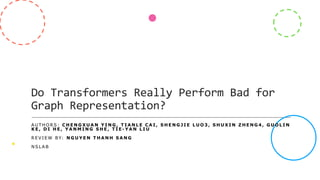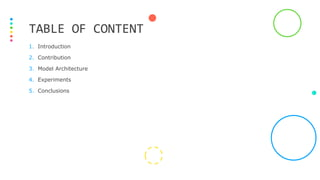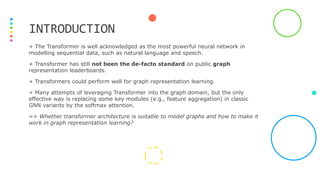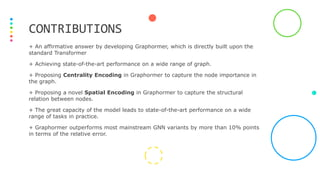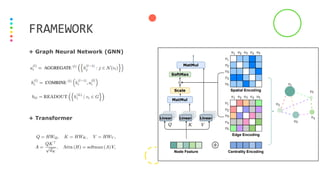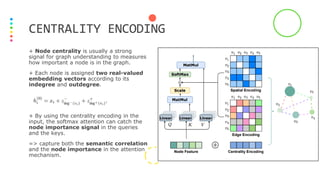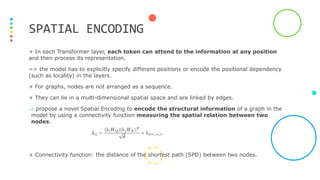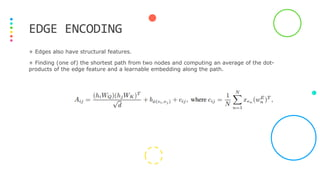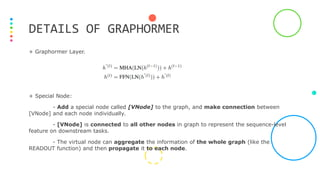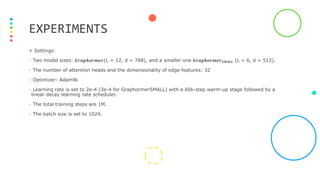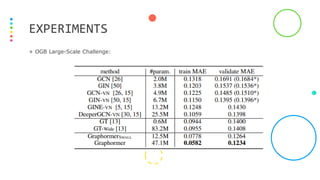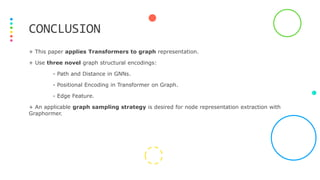1 von 13
Anzeige

### NS-CUK Seminar: S.T.Nguyen, Review on "Do Transformers Really Perform Bad for Graph Representation?," NeurIPS 2021

1. Do Transformers Really Perform Bad for Graph Representation? AU T H O R S : C H E N G X U A N Y I N G , T I A N L E C A I , S H E N G J I E L U O 3 , S H U X I N Z H E N G 4 , G U O L I N K E , D I H E , Y A N M I N G S H E , T I E - Y A N L I U R E V I E W B Y: N G U Y E N T H A N H S A N G N S L A B
2. 1. Introduction 2. Contribution 3. Model Architecture 4. Experiments 5. Conclusions TABLE OF CONTENT
3. + The Transformer is well acknowledged as the most powerful neural network in modelling sequential data, such as natural language and speech. + Transformer has still not been the de-facto standard on public graph representation leaderboards. + Transformers could perform well for graph representation learning. + Many attempts of leveraging Transformer into the graph domain, but the only effective way is replacing some key modules (e.g., feature aggregation) in classic GNN variants by the softmax attention. => Whether transformer architecture is suitable to model graphs and how to make it work in graph representation learning? INTRODUCTION
4. + An affirmative answer by developing Graphormer, which is directly built upon the standard Transformer + Achieving state-of-the-art performance on a wide range of graph. + Proposing Centrality Encoding in Graphormer to capture the node importance in the graph. + Proposing a novel Spatial Encoding in Graphormer to capture the structural relation between nodes. + The great capacity of the model leads to state-of-the-art performance on a wide range of tasks in practice. + Graphormer outperforms most mainstream GNN variants by more than 10% points in terms of the relative error. CONTRIBUTIONS
5. + Graph Neural Network (GNN) + Transformer FRAMEWORK
6. + Node centrality is usually a strong signal for graph understanding to measures how important a node is in the graph. + Each node is assigned two real-valued embedding vectors according to its indegree and outdegree. + By using the centrality encoding in the input, the softmax attention can catch the node importance signal in the queries and the keys. => capture both the semantic correlation and the node importance in the attention mechanism. CENTRALITY ENCODING
7. + In each Transformer layer, each token can attend to the information at any position and then process its representation. => the model has to explicitly specify different positions or encode the positional dependency (such as locality) in the layers. + For graphs, nodes are not arranged as a sequence. + They can lie in a multi-dimensional spatial space and are linked by edges.  propose a novel Spatial Encoding to encode the structural information of a graph in the model by using a connectivity function measuring the spatial relation between two nodes. + Connectivity function: the distance of the shortest path (SPD) between two nodes. SPATIAL ENCODING
8. + Edges also have structural features. + Finding (one of) the shortest path from two nodes and computing an average of the dot- products of the edge feature and a learnable embedding along the path. EDGE ENCODING
9. + Graphormer Layer. + Special Node: - Add a special node called [VNode] to the graph, and make connection between [VNode] and each node individually. - [VNode] is connected to all other nodes in graph to represent the sequence-level feature on downstream tasks. - The virtual node can aggregate the information of the whole graph (like the READOUT function) and then propagate it to each node. DETAILS OF GRAPHORMER
10. + Settings: - Two model sizes: 𝑮𝒓𝒂𝒑𝒉𝒐𝒓𝒎𝒆𝒓(L = 12, d = 768), and a smaller one 𝑮𝒓𝒂𝒑𝒉𝒐𝒓𝒎𝒆𝒓𝑺𝑴𝑨𝑳𝑳 (L = 6, d = 512). - The number of attention heads and the dimensionality of edge features: 32 - Optimizer: AdamW. - Learning rate is set to 2e-4 (3e-4 for GraphormerSMALL) with a 60k-step warm-up stage followed by a linear decay learning rate scheduler. - The total training steps are 1M. - The batch size is set to 1024. EXPERIMENTS
11. + OGB Large-Scale Challenge: EXPERIMENTS
12. + This paper applies Transformers to graph representation. + Use three novel graph structural encodings: - Path and Distance in GNNs. - Positional Encoding in Transformer on Graph. - Edge Feature. + An applicable graph sampling strategy is desired for node representation extraction with Graphormer. CONCLUSION
13. THANK YOU!
Anzeige